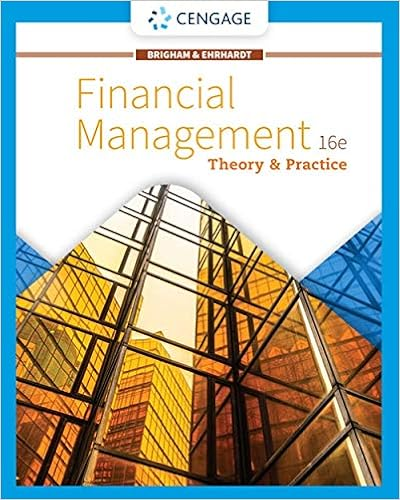# The hedge ratio a is the size of the long short

• Test Prep
• 23
• 84% (37) 31 out of 37 people found this document helpful

This preview shows page 9 - 12 out of 23 pages.

##### We have textbook solutions for you!
The document you are viewing contains questions related to this textbook.The document you are viewing contains questions related to this textbook.
Chapter 8 / Exercise 09
Financial Management: Theory & Practice
Brigham/EhrhardtExpert Verified
56. The hedge ratio A . Is the size of the long (short) position the investor must have in the underlying asset per option the investor must write (buy) to have a risk-free offsetting investment that will result in the investor perfectly hedging the option. B. C. Is related to the number of options that an investor can write without unlimited loss while holding a certain number of shares. D. All of the above.
57. Find the value of a call option written on 100 with a strike price of $1.00 = 1.00. In one period there are two possibilities: the exchange rate will move up by 15% or down by 15% (i.e.$1.15 = 1.00 or $0.85 = 1.00). The U.S. risk-free rate is 5% over the period. The risk-neutral probability of a dollar depreciation is 2 / 3 and the risk-neutral probability of the dollar strengthening is 1 / ##### We have textbook solutions for you! The document you are viewing contains questions related to this textbook.The document you are viewing contains questions related to this textbook. Chapter 8 / Exercise 09 Financial Management: Theory & Practice Brigham/EhrhardtExpert Verified 58. Use the binomial option pricing model to find the value of a call option on 10,000 with a strike price of 12,500. The current exchange rate is 1.50/ 1.00 and in the next period the exchange rate can increase to 2.40/ or decrease to 0.9375/ 1.00 (i.e. u = 1.6 and d = 1/ u = 0.625). The current interest rates are i = 3% and are i = 4%. Choose the answer closest to yours. 59. Find the hedge ratio for a call option on 10,000 with a strike price of 12,500. The current exchange rate is 1.50/ 1.00 and in the next period the exchange rate can increase to 2.40/ or decrease to 0.9375/ 1.00 (i.e. u = 1.6 and d = 1/ u = 0.625). The current interest rates are i = 3% and are i = 4%. Choose the answer closest to yours. 60. You have written a call option on 10,000 with a strike price of$20,000. The current exchange rate is $2.00/ 1.00 and in the next period the exchange rate can increase to$4.00/ 1.00 or decrease to $1.00/ 1.00 (i.e. u = 2 and d = 1/ u = 0.5). The current interest rates are i$ = 3% and are i = 2%. Find the hedge ratio and use it to create a position in the underlying asset that will hedge your option position. A. Buy 10,000 today at $2.00/ 1.00 B. Enter into a short position in a futures contract on 6,666.67 C. Lend the present value of 6,666.67 today at i = 2%. D. Enter into a long position in a futures contract on 6,666.67 E. Both c) and d) would work F. None of the above 61. Draw the tree for a put option on$20,000 with a strike price of 10,000. The current exchange rate is 1.00 = $2.00 and in one period the dollar value of the pound will either double or be cut in half. The current interest rates are i$ = 3% and are i = 2%.
•••